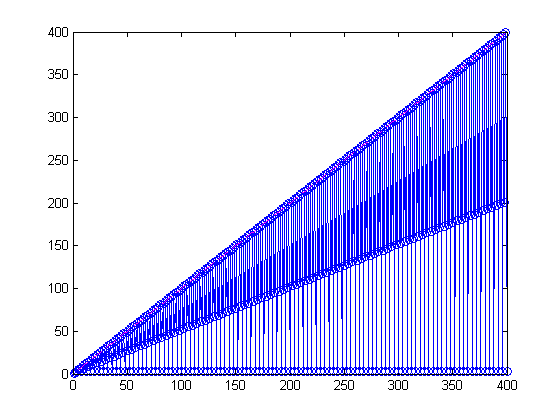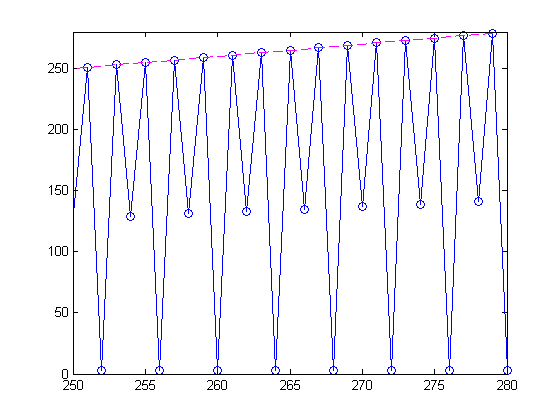# parfor the Course

Starting with release R2007b, there are multiple ways to take advantage of newer hardware in MATLAB. In MATLAB alone, you can benefit from using multithreading,
depending on what kind of calculations you do. If you have access to Distributed Computing Toolbox, you have an additional set of possibilities.

### Problem Set Up

Let's compute the rank of magic square matrices of various sizes. Each of these rank computations is independent of the others.

n = 400;
ranksSingle = zeros(1,n);

Because I want to compare some speeds, and I have a dual core laptop, I will run for now using a single processor using the

maxNumCompThreads(1);
tic
for ind = 1:n
ranksSingle(ind) = rank(magic(ind));
end
toc
plot(1:n,ranksSingle, 'b-o', 1:n, 1:n, 'm--')
Elapsed time is 22.641646 seconds.Zooming in, youI can see a pattern with the odd order magic squares having full rank.

axis([250 280 0 280])Since each of the rank calculations is independent from the others, we could have distributed these calculations to lots of
processors all at once.

### Parallel Version

With Distributed Computing Toolbox, you can use up to 4 local workers to prototype a parallel algorithm. Here's what the
algorithm for the rank calculations. parMagic uses parfor, a new construct for executing independent passes through a loop. It is part of the MATLAB language, but behaves essentially
like a regular for loop if you do not have access to Distributed Computing Toolbox.

dbtype parMagic
1     function ranks = parMagic(n)
2
3     ranks = zeros(1,n);
4     parfor (ind = 1:n)
5         ranks(ind) = rank(magic(ind));  % last index could be ind,not n-ind+1
6     end


### Run Parallel Algorithm and Compare

Let's run the parallel version of the algorithm from parMagic still using a single process and compare results with the original for loop version.

tic
ranksSingle2 = parMagic(n);
toc
isequal(ranksSingle, ranksSingle2)
Elapsed time is 22.733663 seconds.
ans =
1


### Run in Parallel Locally

Now let's take advantage of the two cores in my laptop, by creating a pool of workers on which to do the calculations using
the matlabpool command.

matlabpool local 2
tic
ranksPar = parMagic(n);
toc
To learn more about the capabilities and limitations of matlabpool, distributed
arrays, and associated parallel algorithms, use   doc matlabpool

We are very interested in your feedback regarding these capabilities.

Submitted parallel job to the scheduler, waiting for it to start.
Connected to a matlabpool session with 2 labs.
Elapsed time is 13.836088 seconds.


### Comparison

Did we get the same answer?

isequal(ranksSingle, ranksPar)
ans =
1


In fact, we did! And the wall clock time sped up pretty decently as well, though not a full factor of 2.

Let me close the matlabpool to finish off the example.

matlabpool close
Sending a stop signal to all the labs...
Waiting for parallel job to finish...
Performing parallel job cleanup...
Done.


### Local Workers

With Distributed Computing Toolbox, I can use up to 4 local workers. So why did I choose to use just 2? Because on a dual-core
machine, that just doesn't make lots of sense. However, running without a pool, then using a pool of size 1 perhaps, 2 and,
and maybe 4 helps me ensure that my algorithm is ready to run in parallel, perhaps for a larger cluster next. To do so additionally
requires MATLAB Distributed Computing Engine.

### parfor and matlabpool

matlabpool started 2 local matlab workers in the background. parfor in the current "regular" matlab decided how to divide the parfor range among the 2 matlabpool workers as the workers performed the calculations. To learn a bit more about the constraints of code that works in a parfor loop, I recommend you read the portion of documentation on variable classifications.